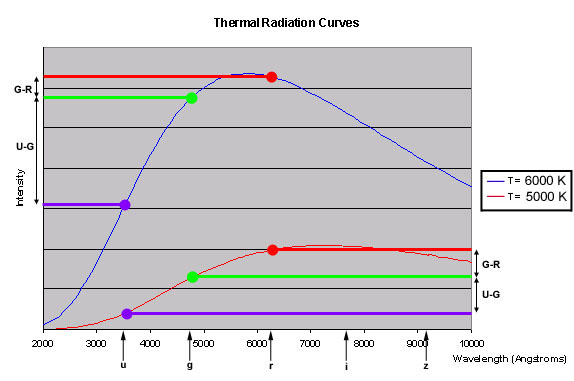A thermal source is an object whose spectrum looks like a perfect thermal radiation curve. A hot plate heating on a stove is a good thermal source. If you look at such a plate through a spectrograph, you will see a curve like the ones you saw in the computer simulation in Explore 2.

So are stars thermal sources? In Explore 4, you started to find out when you looked at the spectra of a few stars in the SDSS. But you can use a color-color diagram to find out exactly what stars are thermal sources and which are not.

To understand how a thermal source should look on a color-color diagram, imagine looking at two thermal sources (they could be anything, but say they’re hot plates heating on a stove). One thermal source is at T = 6000 K (5730 C or 10,340 F) and one is at T = 5000 K (4730 C or 8540 F). The thermal radiation curves are shown below. The blue curve is for the 6000 K thermal source the red curve is for 5000 K thermal source. If you looked at the two thermal sources with the SDSS’s spectrograph, you would see these two curves.If you instead looked at the two thermal sources with the SDSS’s camera, you would see images of the two sources. They would have certain magnitudes and colors. The image above helps you figure out what colors the two sources would have in the images. The colored dots mark the wavelengths of the SDSS’s ultraviolet, green, and red filters. The graph shows how the SDSS’s image gives you three “snapshots” of the thermal radiation curve.

In both curves, the green-wavelength intensity (green dot) is greater than the ultraviolet-wavelength intensity (purple dot). That is, the green magnitude is less than the ultraviolet magnitude. (Because magnitude decreases as the intensity of light emitted by a star increases, magnitude decreases moving up the y-axis on this graph.) So u-g, which is the difference between the ultraviolet and green magnitudes, is positive for both curves, but it is less for the T = 5000 K curve. Similarly, g-r increases between the T = 6000 K curve and the T = 5000 K curve, although not as much as u-g.

Question 4. What would you get if you graphed these two thermal sources on a
u-g/g-r diagram? What do you think you would get if you added more thermal sources (such as T = 4000 K or 8000 K) to this diagram?

As a thermal source’s temperature increases, its thermal radiation curve changes. As the curve changes, the object’s colors also change. Because the SDSS’s filters are always at the same wavelengths, though, if one color (such as u-g) decreases, the next color (g-r) will increase. When you make a color-color diagram for thermal sources of different temperatures, you will get a straight line.

Question 5. Can all the stars in your color-color diagram be considered thermal sources? Why or why not? If not, which stars are not thermal sources?